output.to from Sideway
Number Theory

Prime Number

infinitude of Prime Number

2

Draft for Information Only

# Content

```  Infinitely Many Prime Numbers    Washington's Proof (1980)   Furstenberg's Proof (1955)   Euclidean Sequences    Pairwise Relatively Prime Sequences ```

## Infinitely Many Prime Numbers

"There exist infinitely many prime numbers" as there are infinitely natural numbers on the number line. Many supporting proofs can support the existence of infinitely prime numbers along the number line.

### Washington's Proof (1980)

Washington's method is via commutative algebra. Consider the field of all numbers with the form a+b√(-5) where a, b are rational numbers. Therefore the ring of algebraic integers in this field are numbers of the same form with a, b can only be ordinary integers only. In this ring, 2, 3, 1+√(-5), 1-√(-5) are prime elements bescause these numbers have no other algebraic integer prime factor besides the "unit" 1 or -1. 6 is a composite element in the ring of algebraic integers. And the number 6 can be decomposited into 2x 3 or (1+√(-5))(1-√(-5)). The product of primes of number 6 is not unique up to units, so this ring is not a unique factorization domain. Since the ring of algebraic integers in every number field of finite degree is a Dedekind domain, where every nonideal is the product of prime ideals in a unique way. And for a Dedekind domain with only finitely many prime ideals is a principal ideal domain, where every nonelement is the product of prime elements in a unique way and is up to units. Therefore this ring is not a principal ideal domain and there must have infinitely many prime ideals. And in every number field of finite degree, there are only finitely many prime ideals that divide any given prime number. The infinitely many prime ideals imply there exist infinitely many prime numbers.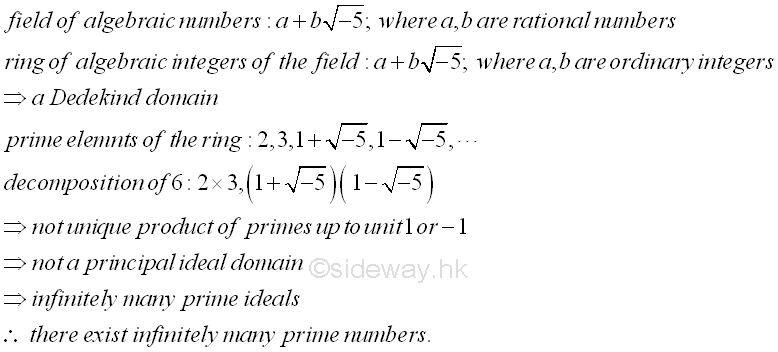### Furstenberg's Proof (1955)

Furstenberg's Method is based on ideas of topology. Considering the set of integers Z={..., -3. -2, -1, 0, 1, 2, 3, ...}, let collection B of subsets of Z be the evenly topological space of integer from -∞ to +∞ of using arithmetic progressions, as a basis for a topology on Z such that every element of Z is contained in at least one basic element. The family of sets B(a,b)={an+b|n∈Z}=aZ+b with a and b are whole number and a not equal to 0, is the set of integers Z. Let x∈Bi, if Bi⊂Z, then x∈Bi⊂Z. Therefore if x∈B1∩B2 for any two basis elements Bi, there exists a basis element B3 such that x∈B3⊂B1∩B2. A topology on the set Z with the collection B of subsets of Z have properties that ∅ and Z are in the collection B. The union set of the basis elements of any finite subcollection of collection B is also in B and the intersection set of the basis elements of any finite subcollection of B is also in B. Therefore, Z is a topological space with collection B. Consider a subset S of Z, set S is an open set of Z if S belongs to the collection B. In order words, the topological space is the set Z with a collection of subsets S of Z. These subsets S of Z are called open sets because one subset S belongs to the collection of all subsets S, for example ∅ and X are both open and the union of arbitrary open sets and the intersection of finite open sets are open also. Similarly to the topology of Z generated by collection B, a subset S of Z is said to be an open set in Z, if for each x∈S, there is a basis element Bi∈B such that x∈Bi and Bi⊂S. Since a set S is called closed if its complement is open. Therefore S is both closed and open because its complement is the union of other subsets S. And imply the union of any finite number of subsets S is closed. Therefore the topological space on Z have properties that ∅ and Z are closed because they are the complements of the open sets Z and ∅.  The arbitary intersection set of the closed sets are closed and the finite unions of closed sets are closed. Since family of sets B(a,b) are basis sets and are equal to the compliement of the union of other basis sets, that is B(a,b)=Z\∪a-1
i=1
B(a,b+j), imply family of sets B(a,b) are closed. Therefore family of sets B(a,b) are both open and closed. And also imply that the union of any finite number of sets B(a,b) is closed. Besides set B(a,b)=aZ+b is infinite, every non empty open set in the topology on Z is infinite, imply no finite set is open. Consider one kind of subset A in the set Z, let set Ap be all multiples of prime p, imply Ap=B(p,0)=pZ and set A is equal to the union of all set Ap where p runs throught the set of primes ≥2, i.e A=∪
p=prime
Ap
. Since A consists all x in set Z except -1 and 1, there exist another set {-1,1} and is the complement of A. That is A=∪
p=prime
Ap=Z\{-1,1}
. The set {-1,1} is finite and is not an open set, imply A cannot be closed. If there exists only finitely many primes, a finite union of closed sets is equal to a closed set which contradicts to the complement of a closed set. Since A is open, A cannot be a finite union of closed sets and can only be an union of infinitely sets imply there are infinitely many prime numbers.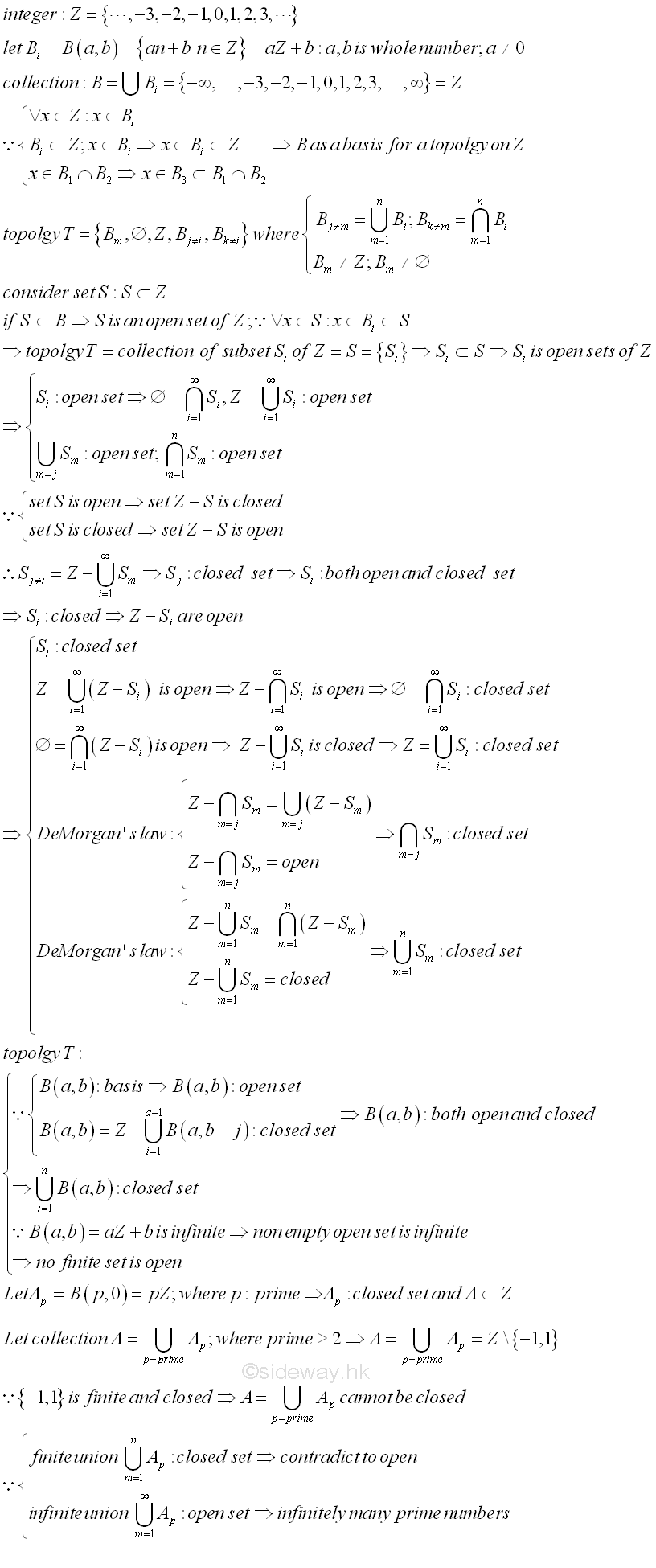### Euclidean Sequences 

Similar to the Euclid's method, equation can be constructed to generate an infinite prime number sequence. Some typical sequences are: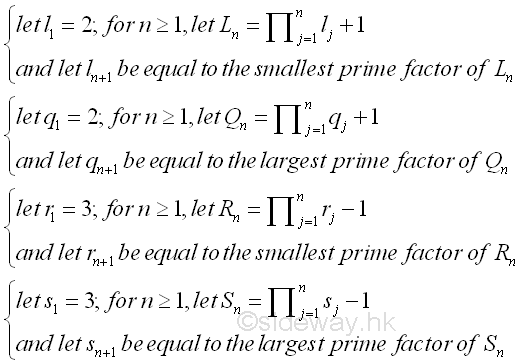These sequences are not monotonic. These sequences do not contain all the primes and have been conjectured that infinitely many  primes are excluded. For example, sequence of Ln is generated as

2*smallest+1 2*largest+1 3*smallest;-1 3*largest;-1
n ln Ln qn Qn rn Rn sn Sn
1 2 2+1 2 2+1 3 3-1 3 3-1
2 3 2*3+1 3 2*3+1 2 3*2-1 2 3*2-1
3 7 2*3*7+1 7 2*3*7+1 5 3*2*5-1 5 3*2*5-1
4 43 2*3*7*43+1 43 2*3*7*43+1 29 3*2*5*29-1 29 3*2*5*29-1
5 13*139 2*3*7*43*13+1 13*139 2*3*7*43*139+1 11*79 3*2*5*29*11-1 11*79 3*2*5*29*79-1
... ... ... ... ... ... ... ... ...

An infinite prime number sequence can also be generated from the sequence of known existing primes. Let pn be the nth of prime number, the product of the first n primes is called the primorial pn#. The two sequences are  pn#+1 and  pn#-1. Imply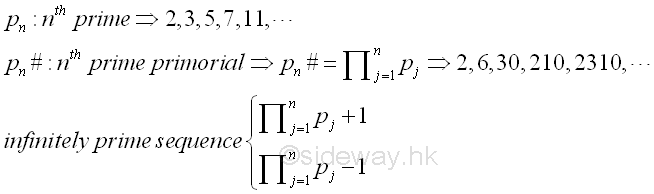Similary, there are infinitely many generated prime only  or composite are still unknown.

n pn pn#+1 pn#-1
1 2 2+1 3 2-1 1
2 3 2*3+1 7 2*3-1 5
3 5 2*3*5+1 31 2*3*5-1 29
4 7 2*3*5*7+1 211 2*3*5*7-1 11*19
5 11 2*3*5*7*11+1 2311 2*3*5*7*11-1 2309
6 13 2*3*5*7*11*13+1 59*509 2*3*5*7*11*13-1 30029
... ... ...   ... ...

### Pairwise Relatively Prime Sequences 

Similar to Goldbach's method of using an infinitely Pairwise Relatively Prime Sequences, Bellman (1947) proposed an induction method to generate infinite sequences of pairwise relatively prime integers. By method of induction, consider a polynomial f(x) with integral coefficients. then let f1(x)=f(x) and define fn+1(x)=f(fn(x)) for n≥1. For f(0)≠0 and n≥2, if f1(0)=f(0) and gcd(m,f(0))=1 such that m and f(0) are relatively prime integers, imply f(m) and f(0) are also relatively prime integers gcd(f(m),f(0))=1. Therefore by induction, let f1(x)=f(x) and for n≥1, let fn+1(x)=f( fn(x)). If fn(0)=f(0) and gcd(m,f(0))=1 then integers m,  f1(m), f2(m), f3(m), ..., fi(m), fn(m), ... are pairwise relatively primes.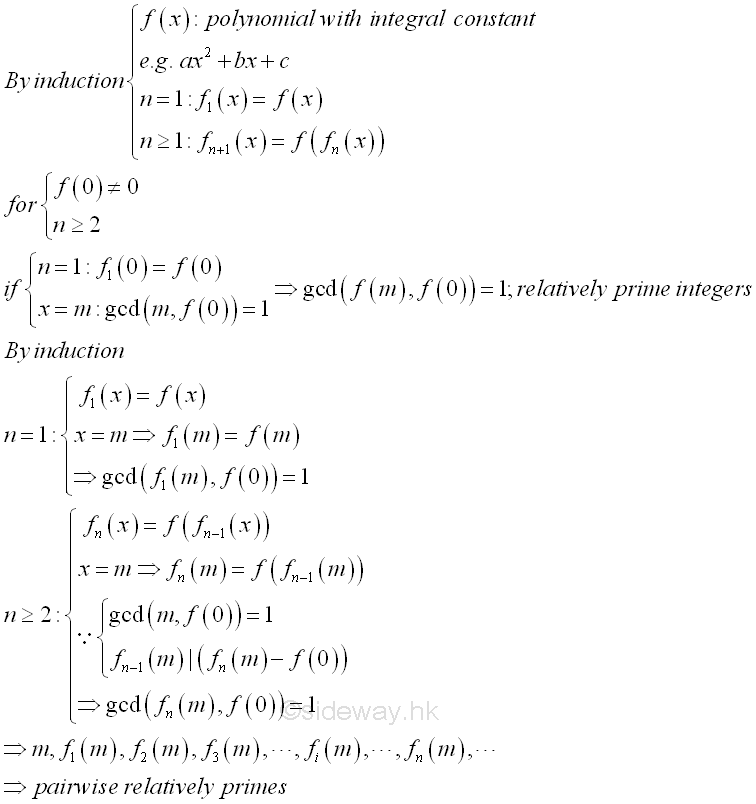There are many particular cases of this theorem.  For example, the Fermat number, Fn=22n+1 can be expressed as a  polynomial f(x) with integral coefficients, f(x)=(x-1)2+1 with x equal to -1. Imply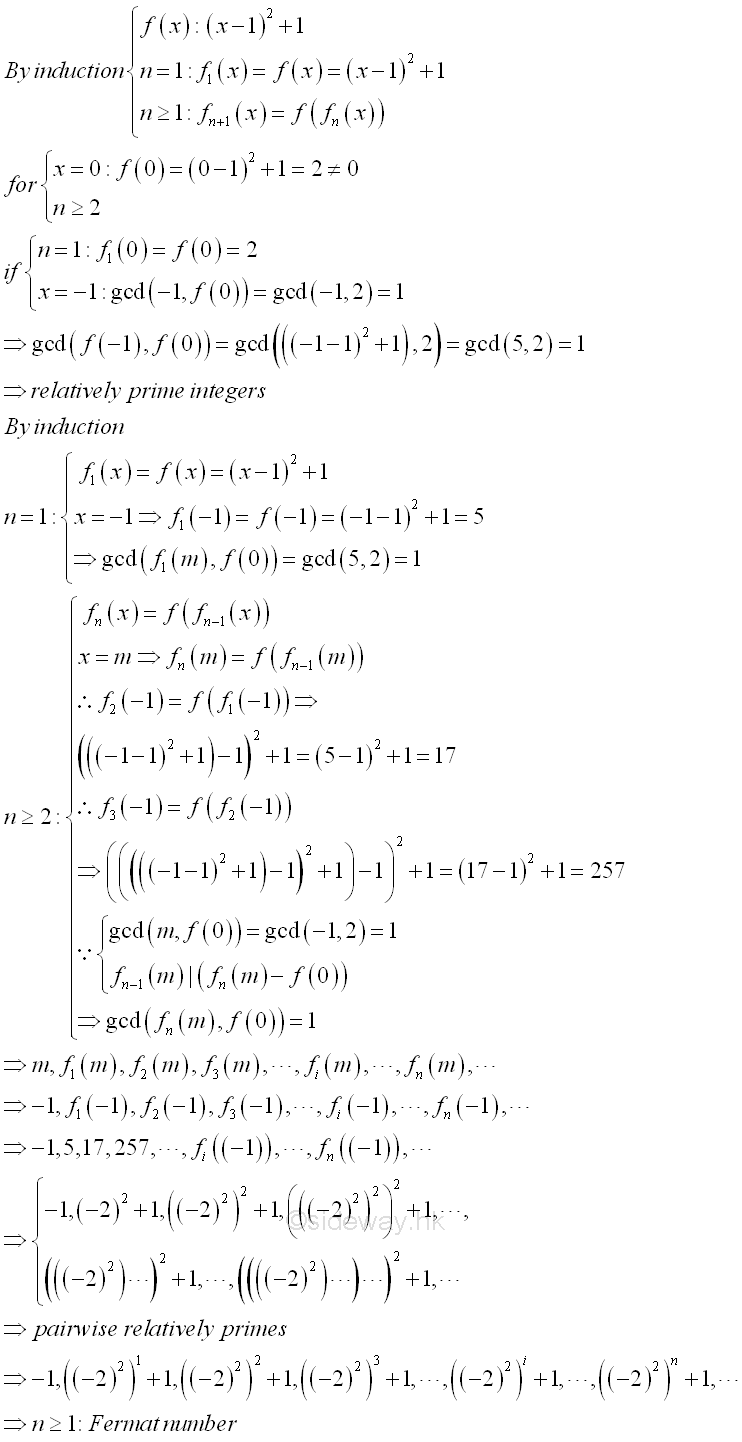Another example is from Edwards (1964) (or Mohanty, 1978), Edwards proposed a sequence generated by a recursive polynomial formula. Let a, S0 be non zero relatively prime integers; for n≥1, Sn is equal to  Sn-1(Sn-1-a)+a. Similarly, S0, S1, S2, S3, ..., Sn, ... are pairwise relatively prime integers. One particular case is when a=2, S0=3, the sequences are S0=3, S1=5, S2=17, S3=257, ..., Sn, ... These sequences are same as the Fermat number sequence where Sn=Fn. In fact, these sequences can alse be obtained using the Bellman's method. Let function fn(x) be Sn. The Edwards's polynomial, Sn=Sn-1(Sn-1-a)+a is same as the Bellman's polynomial fn+1(x)=(fn(x))2-a(fn(x))+a. implies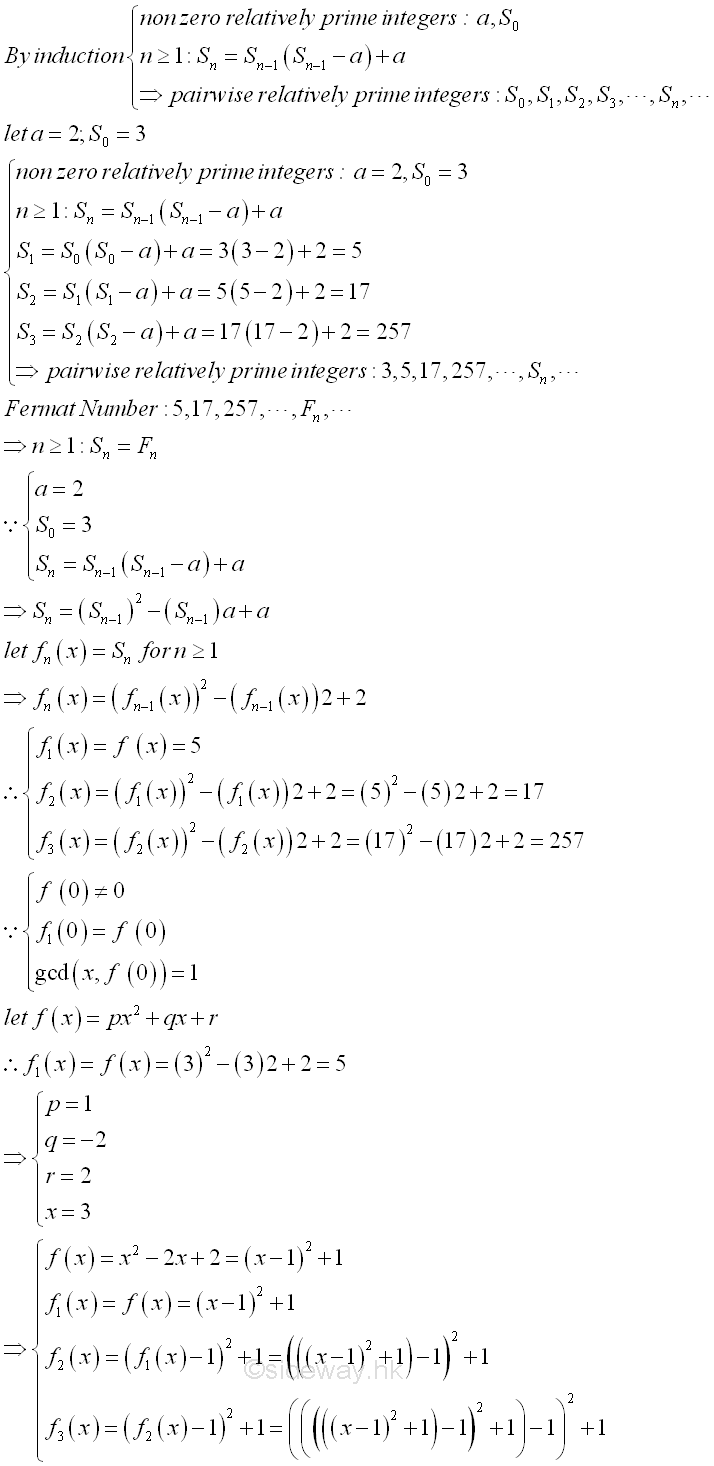Consider another infinite pairwise relatively prime sequences. Let w1=2, w2=w1+1=3, w3=w1w2+1=7,wn+1=∏n
i=1
wi. The numbers w1, w2, w3, ..., wn, ...  are pairwise relatively prime. When a prime pi dividing wi, prime pi is distinct. Therefore primes  p1, p2, p3, ..., pn, ...  are all distinct primes. Similarly, the infinite pairwise relatively prime sequences can be expressed as the Bellman's polynomial fn+1(x)=(fn(x))2-(fn(x))+1. Implies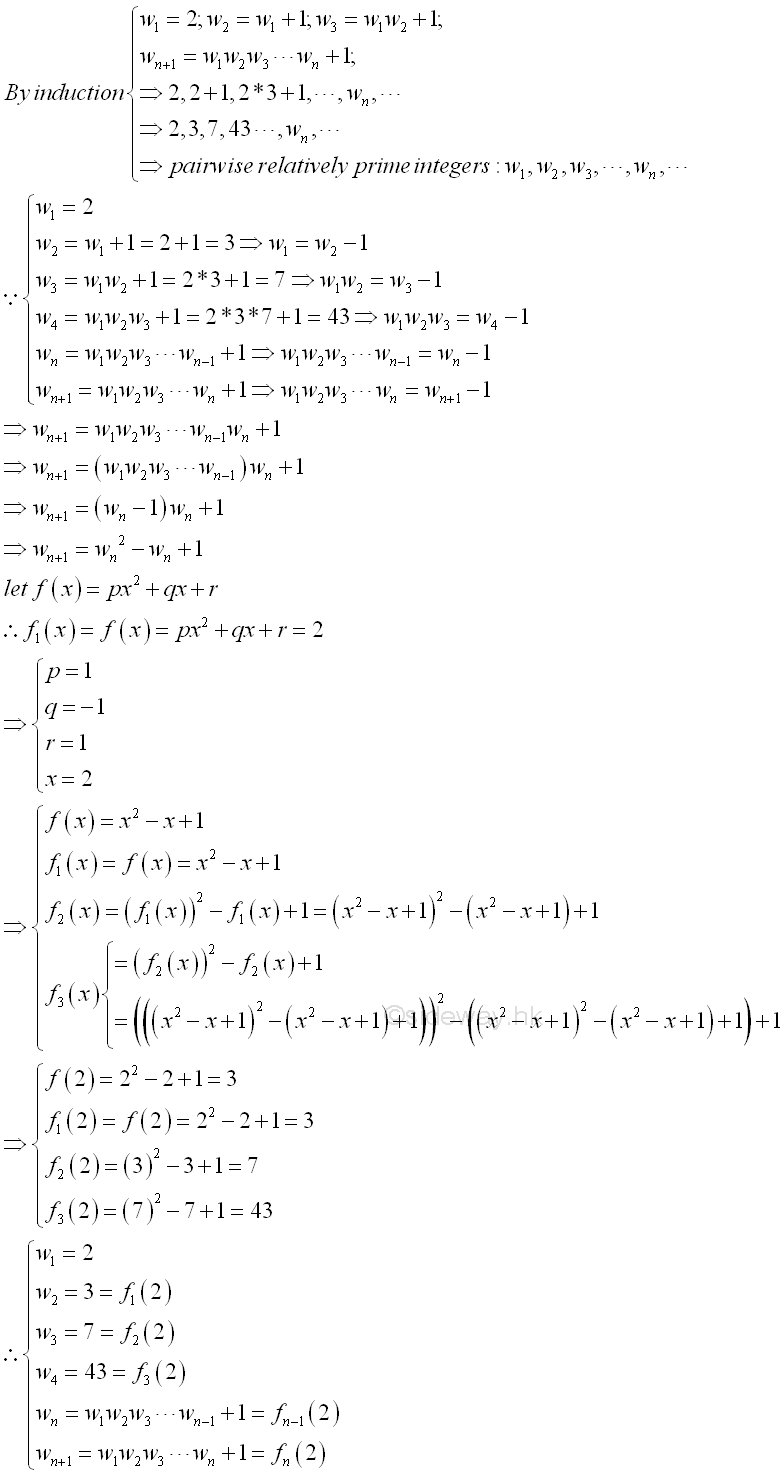©sidewayReferences

1. R. Paulo, 1996, The New Book of Prime Number Records, Springer-Verlag, New York
2. Wolstenholme, R.J., 1862, On Certain Properties of Prime Numbers, The Quarterly Journal of Pure and Applied Mathematics, Vol 5, p35-39, London
3. Mann, H.B., Shanks D., 1972, A Necessary and Sufficient Condition for Primality, and Its Source, Journal of Combinatorial Theory, 13, p131-134
4. J.M Pollard, Kangaroos, 1975, A Monte Carlo Method for Factorization, BIT 15 (1975), 331-334ID: 130400009 Last Updated: 2013/4/16 Revision: Ref:Home (5)

Business

Management

HBR (3)

Information

Recreation

Hobbies (7)

Culture

Chinese (1097)

English (336)

Reference (66)

Computer

Hardware (149)

Software

Application (187)

Digitization (24)

Numeric (19)

Programming

Web (644)CSS (SC)

ASP.NET (SC)

HTML

Knowledge Base

Common Color (SC)

Html 401 Special (SC)

OS (389)MS Windows

Windows10 (SC)

.NET Framework (SC)

DeskTop (7)

Knowledge

Mathematics

Formulas (8)

Number Theory (206)

Algebra (20)

Trigonometry (18)

Geometry (18)

Calculus (67)

Complex Analysis (21)

Engineering

Tables (8)

Mechanical

Mechanics (1)

Rigid Bodies

Statics (92)

Dynamics (37)

Fluid (5)

Control

Acoustics (19)

Biology (1)

Geography (1)

Latest Updated Links

Copyright © 2000-2019 Sideway . All rights reserved Disclaimers last modified on 10 Feb 2019